Home
Hostname: page-component-55597f9d44-mm7gn Total loading time: 0.275 Render date: 2022-08-10T20:55:21.192Z Has data issue: true Feature Flags: { "shouldUseShareProductTool": true, "shouldUseHypothesis": true, "isUnsiloEnabled": true, "useRatesEcommerce": false, "useNewApi": true } hasContentIssue true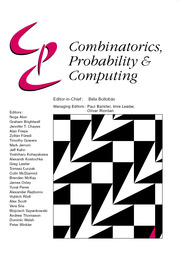Combinatorics, Probability and Computing

# On subgraphs of C2k-free graphs and a problem of Kühn and Osthus

Published online by Cambridge University Press:  04 February 2020

*
*Corresponding author. Email: ctompkins496@gmail.com
*Corresponding author. Email: ctompkins496@gmail.com

## Abstract

Let c denote the largest constant such that every C6-free graph G contains a bipartite and C4-free subgraph having a fraction c of edges of G. Győri, Kensell and Tompkins showed that 3/8 ⩽ c ⩽ 2/5. We prove that c = 38. More generally, we show that for any ε > 0, and any integer k ⩾ 2, there is a C2k-free graph$G'$ which does not contain a bipartite subgraph of girth greater than 2k with more than a fraction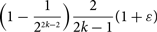$$\Bigl(1-\frac{1}{2^{2k-2}}\Bigr)\frac{2}{2k-1}(1+\varepsilon)$$
of the edges of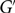$G'$ . There also exists a C2k-free graph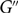$G''$ which does not contain a bipartite and C4-free subgraph with more than a fraction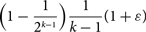$$\Bigl(1-\frac{1}{2^{k-1}}\Bigr)\frac{1}{k-1}(1+\varepsilon)$$
of the edges of$G''$ .

One of our proofs uses the following statement, which we prove using probabilistic ideas, generalizing a theorem of Erdős. For any ε > 0, and any integers a, b, k ⩾ 2, there exists an a-uniform hypergraph H of girth greater than k which does not contain any b-colourable subhypergraph with more than a fraction$$\Bigl(1-\frac{1}{b^{a-1}}\Bigr)(1+\varepsilon)$$
of the hyperedges of H. We also prove further generalizations of this theorem.

In addition, we give a new and very short proof of a result of Kühn and Osthus, which states that every bipartite C2k-free graph G contains a C4-free subgraph with at least a fraction 1/(k−1) of the edges of G. We also answer a question of Kühn and Osthus about C2k-free graphs obtained by pasting together C2l’s (with k >l ⩾ 3).

## MSC classification

Type
Paper
Information
Combinatorics, Probability and Computing , May 2020 , pp. 436 - 454

## Access options

Get access to the full version of this content by using one of the access options below. (Log in options will check for institutional or personal access. Content may require purchase if you do not have access.)

## References

Benson, C. (1966) Minimal regular graphs of girth eight and twelve. Canad. J. Math 18 94.CrossRefGoogle Scholar
Erdős, P. (1967) Gráfok páros körüljárású részgráfjairól (On bipartite subgraphs of graphs, in Hungarian). Mat. Lapok 18 283288.Google Scholar
Erdős, P. and Hajnal, A. (1966) On chromatic number of graphs and set-systems. Acta Math. Hungar. 17 6199.CrossRefGoogle Scholar
Erdős, P. and Simonovits, M. (1982) Compactness results in extremal graph theory. Combinatorica 2 275288.CrossRefGoogle Scholar
Füredi, Z., Naor, A. and Verstraëte, J. (2006) On the Turán number for the hexagon. Adv. Math. 203 476496.CrossRefGoogle Scholar
Győri, E. (1997) C6-free bipartite graphs and product representation of squares. Discrete Math. 165/166 371375.CrossRefGoogle Scholar
Győri, E., Kensell, S. and Tompkins, C. (2016) Making a C 6-free graph C 4-free and bipartite. Discrete Appl. Math. 209 133136.CrossRefGoogle Scholar
Hoeffding, W. (1963) Probability inequalities for sums of bounded random variables. J. Amer. Statist. Assoc. 58 1330.CrossRefGoogle Scholar
Kühn, D. and Osthus, D. (2005) Four-cycles in graphs without a given even cycle. J. Graph Theory 48 147156.CrossRefGoogle Scholar
Lovász, L. (1968) On chromatic number of finite set-systems. Acta Math. Hungar. 19 5967.CrossRefGoogle Scholar
Mirsky, L. (1971) A dual of Dilworth’s decomposition theorem. Amer. Math. Monthly 78 876877.CrossRefGoogle Scholar
Nešetřil, J. and Rödl, V. (1978) On a probabilistic graph-theoretical method. Proc. Amer. Math. Soc. 72 417421.CrossRefGoogle Scholar

# Save article to Kindle

Note you can select to save to either the @free.kindle.com or @kindle.com variations. ‘@free.kindle.com’ emails are free but can only be saved to your device when it is connected to wi-fi. ‘@kindle.com’ emails can be delivered even when you are not connected to wi-fi, but note that service fees apply.

Find out more about the Kindle Personal Document Service.

On subgraphs of C2k-free graphs and a problem of Kühn and Osthus
Available formats
×

# Save article to Dropbox

To save this article to your Dropbox account, please select one or more formats and confirm that you agree to abide by our usage policies. If this is the first time you used this feature, you will be asked to authorise Cambridge Core to connect with your Dropbox account. Find out more about saving content to Dropbox.

On subgraphs of C2k-free graphs and a problem of Kühn and Osthus
Available formats
×

# Save article to Google Drive

To save this article to your Google Drive account, please select one or more formats and confirm that you agree to abide by our usage policies. If this is the first time you used this feature, you will be asked to authorise Cambridge Core to connect with your Google Drive account. Find out more about saving content to Google Drive.

On subgraphs of C2k-free graphs and a problem of Kühn and Osthus
Available formats
×
×